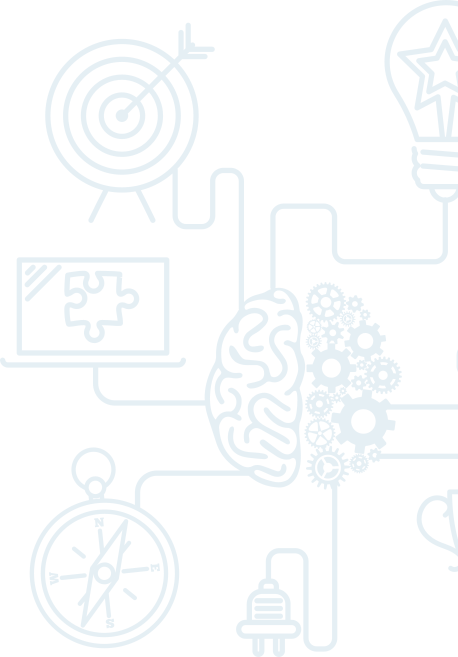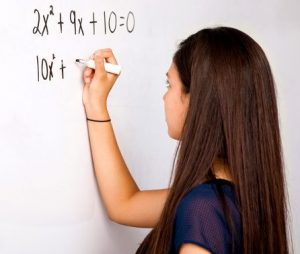# Math Level B (NEW Course)

In response to customer feedback, we have expanded and re-levelled our math program for Fall-Winter 2023-24. As a result, some of our courses have been renamed for 2023-24.Our new Level B Math & Problem-Solving Program targets students in Grades 5 through 7. Students at other grade levels may be placed in Level B to ensure a foundation of mathematical and problem-solving skills.

The major emphasis at this level of program is learning how to think critically and apply problem solving strategies to a variety of problems. Students work with fractions, decimals and percents and build an understanding of integers and rational numbers.

The workbook uses problem solving to place the focus on the student to discover and explore mathematical concepts in context. Students learn to think abstractly, collaborate with others, persevere, and approach problems from different perspectives.

Lessons include interactive puzzles, brain teasers, logic problems, and competitions to help students stay sharp, discover new strategies, and develop mathematical thinking through numerous approaches.

Level B instruction includes, but is not limited to:

Math

• Advanced place value and base ten patterns
• Advanced fractions and application of fraction operations
• Integers
• Operations with rational numbers
• Understanding percent and calculating percents, fractions, decimals
• Ratios and rates
• Application of proportions, scale and similar figures
• Percent proportion and percent change
• Expressions and simple equations
• The coordinate plane
• Understanding slope
• Function tables and graphs

Strategy games & logic puzzles

• Magic squares
• Crack the code
• Find the mystery number
• Sudoku
• Logic charts
• Optical illusions
• Competitions
• Games and development
• Card games and puzzles

Memory development

• Brain Power memory technique

Logic and Problem-Solving Strategies

• 4-step problem solving
• Modeling problems, draw a picture, use a diagram
• Forward solving strategies
• Backwards solving strategies
• Using Venn diagrams
• Logical charts with multiple relationships
• Systematic list
• Organize data
• Problem decomposition
• Encryption and decoding
• Investigating patterns
• Review and check

Prerequisite for Level B: ease of whole number operations (+,-,x,/) and good reading skills.